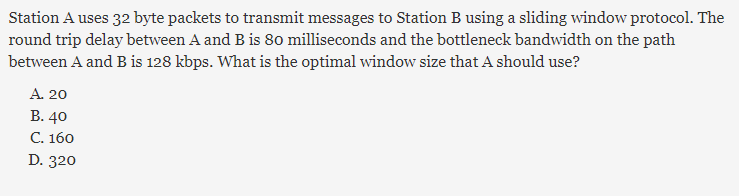##### Find the optimal window size in sliding window.shivani
6 Sep 2017 12:20 pm

Following things are given:
L=32B
TP = 40ms since , RTT =2*Tp
B = 128Kbps
Tt = L/B =32*8/128 = 2ms
a= Tp / Tt = 40/2 = 20
window size = 1+2a = 1+2*20 = 41 //here, 1 is added to avoid duplicate packets which is optional , so oprimal window can be 2*a
so, ans is 40

set2018
9 Sep 2017 01:16 pm

but optimal window size in case of sliding window is 1+ 2a ? why u eliminate 1 pls clear this concept

see if GBN window size = 2 ^m -1 receiver window size =1

SR=2^(m-1)(sender window size ) ,also receiver window size will be same

pls clear this

shivani
25 Sep 2017 04:39 pm

u r right , but here we have to go for closest option matching

set2018
25 Sep 2017 04:46 pm

yes i agree but i want to know is there any criteria for choosing optimal.becoz according to forouzon 1+2a is optimal

Preeti Singh
17 Dec 2017 08:33 pm

What will we do in case of numerical type ques ......40 or 41?????